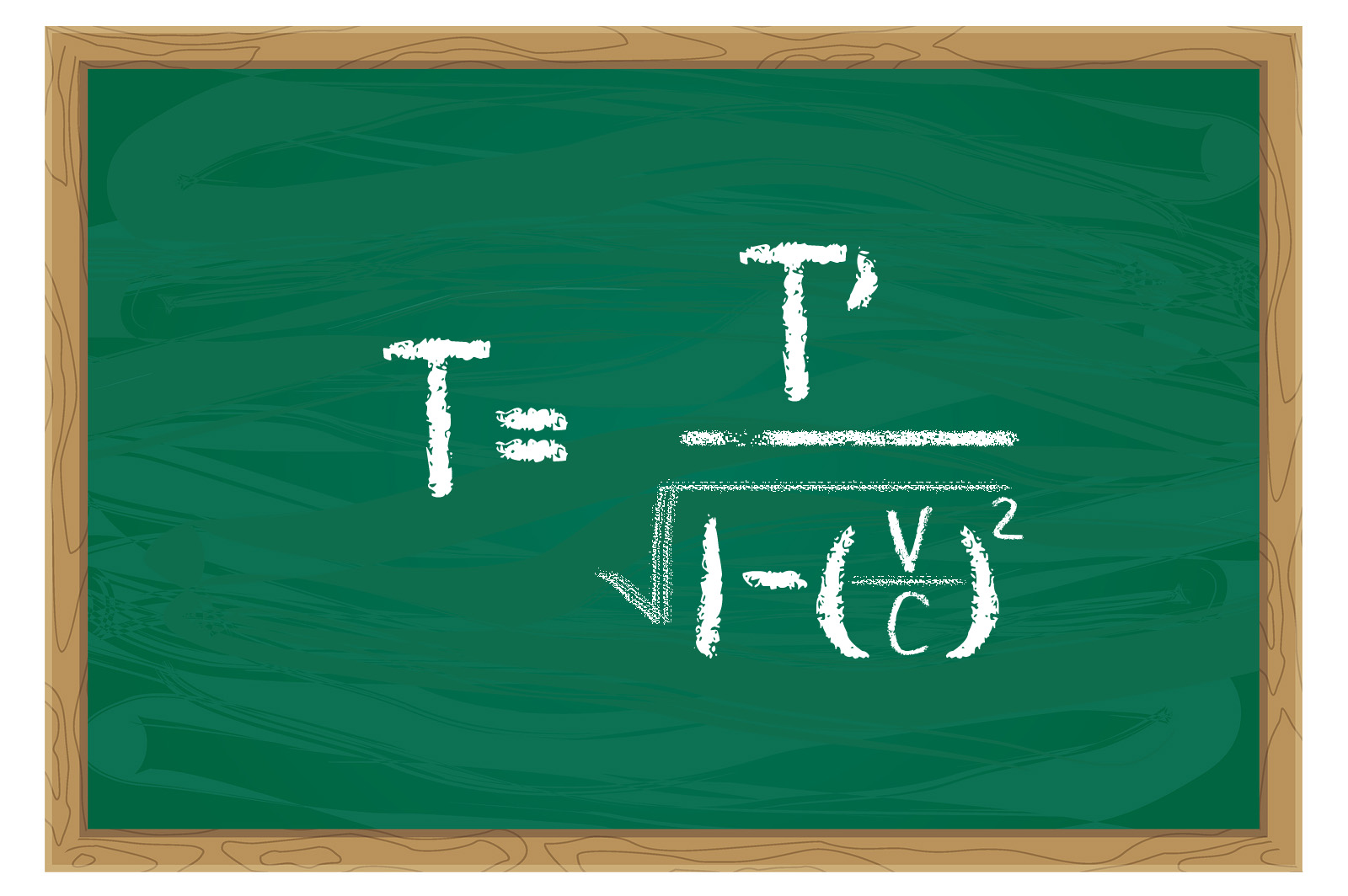# Being TimelessBeing outside of time or timeless is a new concept to science. In classical physics, time is perhaps the most fundamental standard against which everything else is based. When we measure velocity, it’s basic unit is length per second or hour. Feet per second or miles per hour are familiar to most of us. When we measure acceleration, the units are meters per second squared. Newton’s Second Law defines force as mass multiplied by acceleration, so time even becomes involved in parameters that don’t directly include motion.

One of the equations that Einstein gave us which high school students like to play with is the equation for time at very high speeds. For a given frame of reference, time is defined by the equation T = T’/the square root of 1 – V^2 over C^2. In the equation, T = the time you experience. T’ is the time you would experience at rest relative to a given frame of reference. V is the speed at which you move, and C is the speed of light. Notice that as your speed reaches the speed of light, the fraction V^2/C^2 become 1, and since it is subtracted from 1, the value of the denominator becomes zero. Time ceases to exist. It becomes undefined.

If you could exceed the speed of light, the denominator would become the square root of a negative number which is said to be imaginary. Einstein gave us a similar equation for mass in which the mass is the undefined factor. Nuclear physics and quantum mechanics verify these equations.

This is an over-simplified explanation, but the point I am making is that there is physical evidence that there are dimensions beyond the three in which we exist. Being timeless is something science has begun to comprehend because these equations show that time is a variable that can be changed. The creation is far grander and far more mysterious than we can imagine.

For Christians, this is no surprise. The Bible is full of descriptions that embrace the idea that time is a created entity and that creations exist outside of time. The whole concept of there being a beginning to the cosmos in Genesis 1:1 recognizes that there was an existence before time. Proverbs 8:22-23 indicates that time was a designed and fashioned quantity. Revelation 22:13 repeats this concept. Second Timothy 1:9 refers to it, and the whole idea of eternity relies upon it.

When we reach the end of life, time ceases to exist for us. That has interesting implications. If there is no time, there is no death because death depends upon aging, and without time, nothing ages. There is no physical pain if time doesn’t exist. That’s because physical pain depends upon the time it takes for the pain signal to go from what gets hurt (like a finger) to what registers the hurt – your brain.

“No more tears, no more sorrows.” Revelation 21:4 Describes this change saying that “the former things have all passed away.” Second Peter 3:8-13 speaks of the elements dissolving at the end of time. Science has given us some reinforcement for these promises recognizing that the physical world will be done away with when time ends. Let us live in such a way that we can look forward to the timeless new order God has in store for us.
— John N. Clayton © 2019